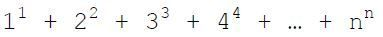Power Function in PythonPosted by Samath3623 March 02, 2015

Write a function power in python (not recursive) that raises an integer n to its nth power and returns that value. If n is less than or equal to 0 then the function should return 0.

e.g.
power(3) = 3**3 = 27
power(4) = 4**4 = 256

Code:

```import math

def power(n):
if(n<=0):
return 0
else:
return math.pow(n,n)```

Write a recursive function sumSeries (using the power function from part 1 ) which computes the following value for an input integer n:If n is less than or equal to 0 return 0

Code:

```def sumSeries(n):
if(n<=0):
return 0
else:
return power(n)+sumSeries(n-1)```